## How the heck do I solve this? Please help T^T A basketball is thrown upward from a height of 1.8 m at a velocity of 12 m/s. How long u

Question

A basketball is thrown upward from a height of 1.8 m at a velocity of 12 m/s. How long until it reaches the ground?

(Recall: h(t)=-4.9t^2-v_0 t+h_0, where v_0 is initial velocity and h_0 is initial height.)

in progress 0
4 months 2021-08-09T12:32:32+00:00 1 Answers 3 views 0

Step-by-step explanation: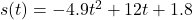where s(t) is the height of the ball after a certain amount of time goes by. If we are looking for how long til it hits the ground, we know 2 things from that question. First we know that the height of an object on the ground is 0, and we also know that t is the time that the ball is in the air. Because the height of the ball when it’s on the ground is 0, we will set s(t) equal to 0 and factor the quadratic to solve for t, the time the ball is in the air. Throw it into the quadratic formula to find those times.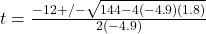which will simplify to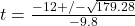The 2 solutions we get from that are that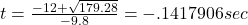and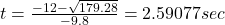Now, the 2 things in math that will never be negative are distances and time, so we will disregard the negative time and go with t = 2.59 seconds. Not sure to where you need to round.